### IMO Shortlist 2012 problem G8

Kvaliteta:
Avg: 0,0
Težina:
Avg: 9,0
Let$ABC$ be a triangle with circumcircle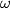$\omega$ and$l$ a line without common points with$\omega$. Denote by$P$ the foot of the perpendicular from the center of$\omega$ to$l$. The side-lines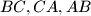$BC,CA,AB$ intersect$l$ at the points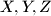$X,Y,Z$ different from$P$. Prove that the circumcircles of the triangles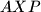$AXP$,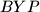$BYP$ and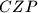$CZP$ have a common point different from$P$ or are mutually tangent at$P$.
Izvor: Međunarodna matematička olimpijada, shortlist 2012